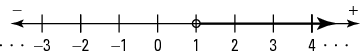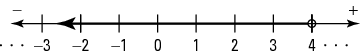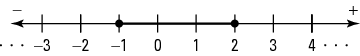## Graphing on a Number Line

Integers and real numbers can be represented on a number line. The point on this line associated with each number is called the graph of the number. Notice that number lines are spaced equally, or proportionately (see Figure 1).

Figure 1. Number lines.#### Graphing inequalities

When graphing inequalities involving only integers, dots are used.

##### Example 1

Graph the set of x such that 1 ≤ x ≤ 4 and x is an integer (see Figure 2).

{ x:1 ≤ x ≤ 4, x is an integer}

Figure 2. A graph of {x:1 ≤ x ≤ 4, x is an integer}.When graphing inequalities involving real numbers, lines, rays, and dots are used. A dot is used if the number is included. A hollow dot is used if the number is not included.

##### Example 2

Graph as indicated (see Figure 3).

1. Graph the set of x such that x ≥ 1.

{ x: x ≥ 1}

2. Graph the set of x such that x > 1 (see Figure 4).

{ x: x > 1}

3. Graph the set of x such that x < 4 (see Figure 5).

{ x: x < 4}

This ray is often called an open ray or a half line. The hollow dot distinguishes an open ray from a ray.

Figure 3. A graph of { x: x ≥ 1}.Figure 4. A graph of { x: x > 1}Figure 5. A graph of { x: x < 4}#### Intervals

An interval consists of all the numbers that lie within two certain boundaries. If the two boundaries, or fixed numbers, are included, then the interval is called a closed interval. If the fixed numbers are not included, then the interval is called an open interval.

##### Example 3

Graph.

1. Closed interval (see Figure 6).

{ x: –1 ≤ x ≤ 2}

2. Open interval (see Figure 7).

{ x: –2 < x < 2}

Figure 6. A graph showing closed interval { x: –1 ≤ x ≤ 2}.Figure 7. A graph showing open interval { x: –2 < x < 2}.If the interval includes only one of the boundaries, then it is called a half‐open interval.

##### Example 4

Graph the half‐open interval (see Figure 8).

{ x: –1 < x ≤ 2}

Figure 8. A graph showing half‐open interval { x: –1 < x ≤ 2}.